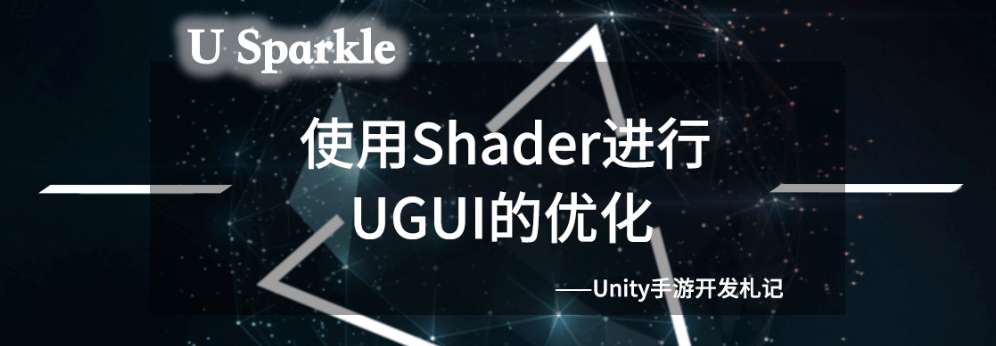## 二、优化目标

• 降低Draw Call
• 减少Overdraw
• 减少UI Mesh重建次数和范围

## 三、小地图

• Overdraw特别大，几乎很多时候会有整个屏幕的Overdraw；
• 玩家在移动过程中，因为一直在持续移动图片的位置（做了适当的降频处理），所以会一直有UI的Mesh重建过程。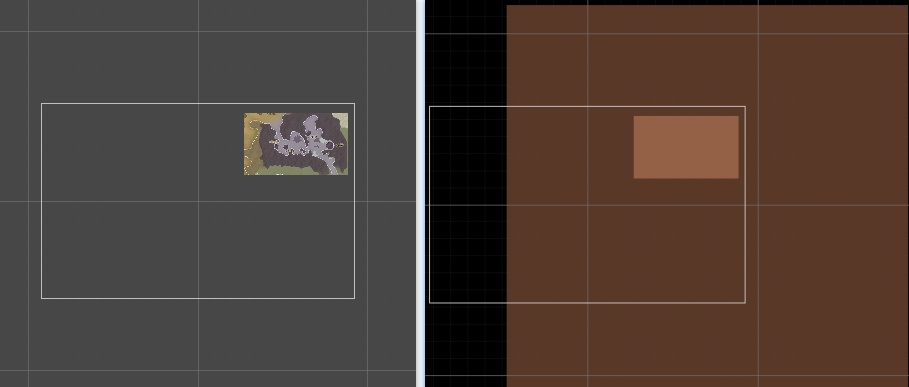``````sampler2D _MainTex;
fixed4 _UVScaleOffset;
sampler2D _BlendTexture;

v2f vert(appdata_t IN)
{
v2f OUT;
OUT.vertex = mul(UNITY_MATRIX_MVP, IN.vertex);
OUT.texcoord = IN.texcoord;
//计算uv偏移
OUT.offsetcoord.xy = OUT.texcoord.xy * _UVScaleOffset.zw + _UVScaleOffset.xy;
#ifdef UNITY_HALF_TEXEL_OFFSET
OUT.vertex.xy -= (_ScreenParams.zw-1.0);
#endif
return OUT;
}

fixed4 frag(v2f IN) : SV_Target
{
half4 color = tex2D(_MainTex, IN.offsetcoord);
half4 blendColor = tex2D(_BlendTexture, IN.texcoord);
color.rgb = blendColor.rgb + color.rgb * (1 - blendColor.a);
return color;
``````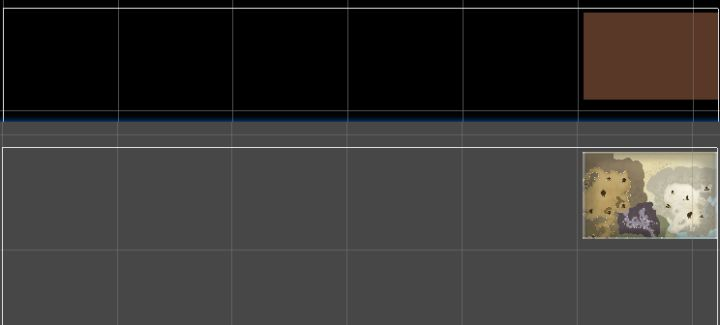• 在那篇文章的最后提到，我们自己拷贝了一个ThorImage类，开放部分接口然后继承。我们后来改成了从Image直接继承的方式，否则之前编写的游戏逻辑要在代码上兼容两种Image，会比较烦，这些向前兼容的需求也是在优化过程中需要额外考虑和处理的点。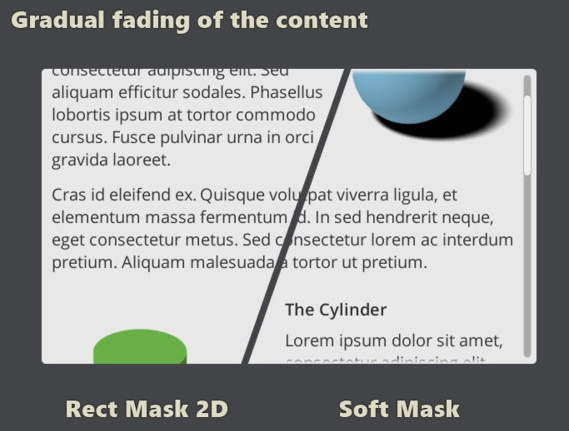## 五、基于DoTween的动画效果优化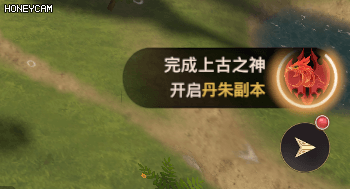``````v2f vert(appdata_t IN)
{
v2f OUT;
OUT.vertex = mul(UNITY_MATRIX_MVP, IN.vertex);
OUT.texcoord = IN.texcoord;

OUT.texcoord.xy -= 0.5;
//避免时间过长的时候影响精度
half t = fmod(_Time.y, 2 * UNITY_PI);
t *= _RotationSpeed;
half s, c;
sincos(t, s, c);
half2x2 rotationMatrix = half2x2(c, -s, s, c);
OUT.texcoord.xy = mul(OUT.texcoord.xy, rotationMatrix);
OUT.texcoord.xy += 0.5;

#ifdef UNITY_HALF_TEXEL_OFFSET
OUT.vertex.xy -= (_ScreenParams.zw-1.0);
#endif
OUT.color = IN.color;
return OUT;
}
``````

Tips：注意所使用贴图的边缘处理，因为UV的旋转可能会导致超过之前0和1的范围。首先贴图的采样方式要使用Clamp的方式，其次贴图的边缘要留出几个像素的透明区域，保证即使在设备上贴图压缩之后依然可以让边缘的效果正确。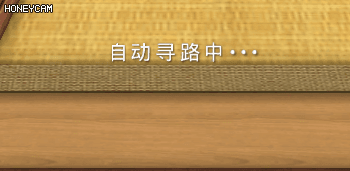``````v2f vert(appdata_t IN)
{
v2f OUT;
UNITY_SETUP_INSTANCE_ID(IN);
UNITY_INITIALIZE_VERTEX_OUTPUT_STEREO(OUT);

//根据时间和配置参数对顶点进行缩放操作
OUT.worldPosition = IN.vertex;
half t = fmod((_Time.y - _TimeOffset) * _TimeScale, 1);
t = 4 * (t - t * t);
OUT.worldPosition.xy -= _VertexParmas.xy;
OUT.worldPosition.xy *= (1 + t*_ScaleRatio);
OUT.worldPosition.xy += _VertexParmas.xy;
OUT.vertex = UnityObjectToClipPos(OUT.worldPosition);

OUT.texcoord = IN.texcoord;

OUT.color = IN.color * _Color;
return OUT;
}
``````

Tips：这里使用UWA群里一位朋友之前提供的三角函数近似的方法来略微减少一下指令消耗：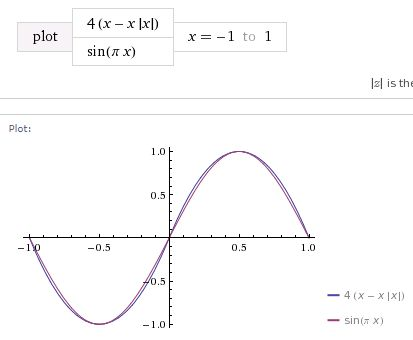sin函数的近似模拟

1）逐个缩放的效果需要控制“自动寻路中...”这句话中的每一个字，因此在这个效果中，每一个字都是一个Text控件。正在缩放的字使用特殊的缩放材质来绘制，其他的字依然使用默认的UI材质绘制，这意味着需要2Draw Call来实现整体效果。

2）vert函数中需要获取字体的中心点和长宽大小，然后进行缩放计算，也就是参数_VertexParmas的内容。经过测试，在UGUI中，顶点的位置信息worldPosition是其相对于Canvas的位置信息，因此这里需要在C#中进行计算，计算过程借助RectTransformUtility的ScreenPointToLocalPointInRectangle函数：

``````Vector2 tempPos;
RectTransformUtility.ScreenPointToLocalPointInRectangle(ParentCanvas.transform as RectTransform,
ParentCanvas.worldCamera.WorldToScreenPoint(img.transform.position),
ParentCanvas.worldCamera, out tempPos);
``````

Tips：这里，搞清楚了vs中顶点的worldPosition对应的属性之后，可以做很多有趣的事情，包括之前的旋转效果也可以不再旋转UV，而是对顶点位置进行旋转。

``````using System;
using System.Collections.Generic;
using UnityEngine;
using UnityEngine.EventSystems;
using UnityEngine.UI;
using DG.Tweening;
using ThorUtils;
using System.Collections;

namespace KEngine.UI
{
public class UIAutoExpand : MonoBehaviour
{
public float ScaleDuration = 1;
public float CycleInterval = 5;
public float ScaleRatio = 0.5f;
private Canvas ParentCanvas;

private Image[] images;
private Vector4[] meshSizes;
private int currentScaleIdx = -1;

private Coroutine playingCoroutine;

private static Material UIExpandMat = null;
private static int VertexParamsID = -1;
private static int TimeOffsetID = -1;
private static int TimeScaleID = -1;
private static int ScaleRatioID = -1;

void Awake()
{
//初始化静态变量
if (UIExpandMat == null)
{
}

UIExpandMat.SetFloat(TimeScaleID, 1 / ScaleDuration);
UIExpandMat.SetFloat(ScaleRatioID, ScaleRatio);

if (ParentCanvas == null)
{
Transform trans = transform;
while(trans != null)
{
ParentCanvas = trans.GetComponent<Canvas>();
if (ParentCanvas != null)
{
break;
}
trans = trans.parent;
}
}
if (ParentCanvas == null || ParentCanvas.worldCamera == null)
{
Debug.LogError("**The parent canvas of UIAutoExpand could not be empty!**");
return;
}
images = GetComponentsInChildren<Image>();
if (images.Length > 0)
{
meshSizes = new Vector4[images.Length];
}
Vector2 tempPos;
for (int i = 0; i < images.Length; ++i)
{
Image img = images[i];
tempPos.x = 0;
tempPos.y = 0;
RectTransformUtility.ScreenPointToLocalPointInRectangle(ParentCanvas.transform as RectTransform,
ParentCanvas.worldCamera.WorldToScreenPoint(img.transform.position),
ParentCanvas.worldCamera, out tempPos);
meshSizes[i].x = tempPos.x;
meshSizes[i].y = tempPos.y;
meshSizes[i].z = 0;
meshSizes[i].w = 0;
}
}

private void OnEnable()
{
playingCoroutine = StartCoroutine(PlayExpandAni());
}

private void OnDisable()
{
if (playingCoroutine != null)
{
StopCoroutine(playingCoroutine);
}
}

private void OnDestroy()
{
StartScaleImage(-1);
if (playingCoroutine != null)
{
StopCoroutine(playingCoroutine);
}
}

private void StartScaleImage(int idx)
{
if (images == null)
{
return;
}
if (currentScaleIdx > -1 && currentScaleIdx < images.Length)
{
Image preImg = images[currentScaleIdx];
preImg.material = null;
}
if (idx < 0 || idx >= images.Length)
{
return;
}
currentScaleIdx = idx;
Image curImg = images[idx];
UIExpandMat.SetVector(VertexParamsID, meshSizes[idx]);
curImg.material = UIExpandMat;
}

private IEnumerator PlayExpandAni()
{
while (true)
{
for (int i = 0; i < images.Length; ++i)
{
StartScaleImage(i);
yield return Yielders.GetWaitForSeconds(ScaleDuration);
}
StartScaleImage(-1);
yield return Yielders.GetWaitForSeconds(CycleInterval);
}
}
}
}
``````

## 六、进度条

UGUI的进度条控件功能非常通用，但是层次很复杂，包括Background、Fill Area和Handle Slide Area三个部分。它是实现原理是基于Mesh的修改：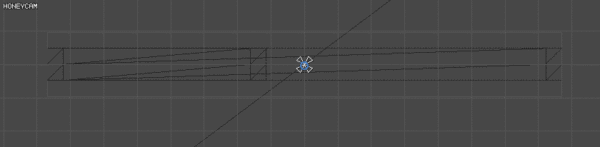Slider控件的更新过程

``````fixed4 frag(v2f IN) : SV_Target
{
half4 color = (tex2D(_MainTex, IN.texcoord) + _TextureSampleAdd) * IN.color;

#if _ISVERTICAL_ON
float uvValue = IN.texcoord.y - _UVRect.y;
float totalValue = _UVRect.w;
#else
float uvValue = IN.texcoord.x - _UVRect.x;
float totalValue = _UVRect.z;
#endif

#if _ISREVERSE_ON
uvValue = totalValue - uvValue;
#endif

color.a *= uvValue / totalValue < _Progress;

color.a *= UnityGet2DClipping(IN.worldPosition.xy, _ClipRect);
#ifdef UNITY_UI_ALPHACLIP
clip (color.a - 0.001);
#endif

return color;
}
``````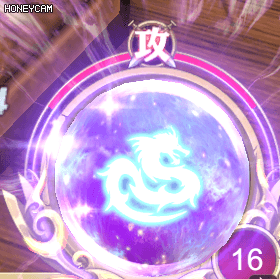``````fixed4 frag(v2f IN) : SV_Target
{
half4 color = (tex2D(_MainTex, IN.texcoord) + _TextureSampleAdd) * IN.color;
float theta = atan2((IN.texcoord.y - _UVRect.y)/_UVRect.w - 0.5,
(IN.texcoord.x + 1E-18 - _UVRect.x)/_UVRect.z-0.5);

#ifdef IS_SYMMETRY
color.a *= ((1 - _Progress) * UNITY_PI < abs(theta));
#else
color.a *= ((1 - _Progress) * UNITY_PI * 2 < theta + UNITY_PI);
#endif

color.a *= UnityGet2DClipping(IN.worldPosition.xy, _ClipRect);
#ifdef UNITY_UI_ALPHACLIP
clip(color.a - 0.001);
#endif

return color;
}
``````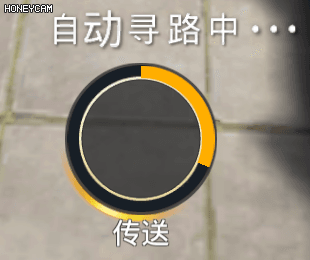UGUI针对Image提供了Filled的Image Type来做环形进度条的效果，其原理是根据角度来更改Mesh实现。

``````/// <summary>
/// 更新贴图的uv值到材质中
/// 注意：需要在Image更新的时候调用本逻辑
/// </summary>
public void UpdateImageUV()
{
if (relativeImage != null)
{
Vector4 uv = (relativeImage.overrideSprite != null) ?
DataUtility.GetOuterUV(relativeImage.overrideSprite) : defaultUV;
uv.z = uv.z - uv.x;
uv.w = uv.w - uv.y;
relativeImage.material.SetVector(UVRectId, uv);
}
}
``````